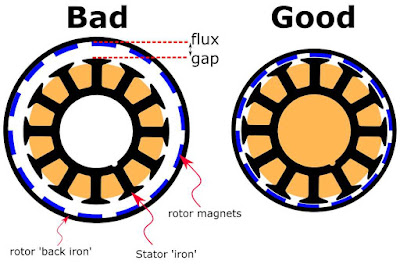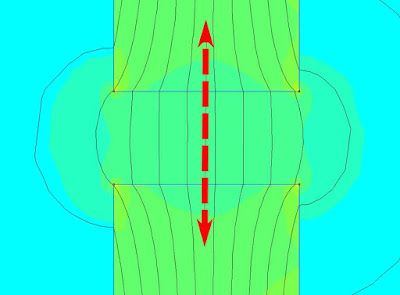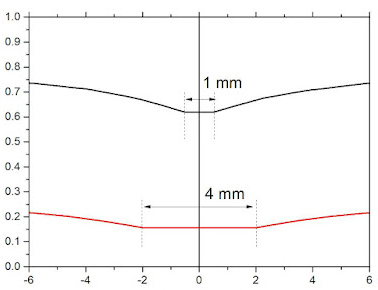## Understanding Brushless Motors - Optimal Flux Gap for High Torque Density

NEWS    |    Nov 18, 2022

What is an air gap?

A simple way to define air gap is to say it is the air in the space between the stator and the rotor of the motor.

More specifically, we can have similar definitions for alternating current or AC motors and direct current or direct current motors.

In an AC motor, the air gap is the air space between the rotor core and the stator. On the other hand, it is said to be the space between the poles and the armature of a DC motor.

The flux gap is the distance between the high permeability material in the stator (stator "iron") and the corresponding high permeability material in the rotor (rotor "back iron"). This material is usually made from thin laminations of Fe-Si steel.

As we all know, in general, the flux gap should be as small as possible.

Note that the flux gap includes magnets. Rare earth magnets (magnetized or unmagnetized) have essentially the same permeability as air. Therefore, from the stator point of view, the magnets are indistinguishable from air and should be included as part of the flux gap.How does the air gap work?

To understand how air gaps work, first remember that electric motors and generators are rotating electrical machines.

This means that electric motors and generators work very similarly. The difference is that electric motors convert the supplied electrical energy into mechanical energy. Instead, generators take specific mechanical energy and convert it into electrical energy.

In both cases, the energy conversion process occurs when the stator and rotor work together to generate magnetic flux through their own copper windings. This is where the air gap comes into play.

A magnetic field is formed in the air gap, and one of the above-mentioned windings is responsible for generating the magnetic flux, which has to pass through the air gap twice for each pole of each phase of the motor.

Some of the key factors related to air gap operation are:

Magnetic force is inversely proportional to the square of the distance. As the air gap size increases, the magnetic attraction decreases and becomes more difficult to control.

Increasing the size of the air gap increases the magnetizing current, which represents the amount of current required to drive magnetic flux through the air gap.

The more poles a motor or generator has, the more times the flux must cross the air gap per revolution.

All these factors lead us to conclude that the smaller the air gap, the better. However, a smaller air gap means less separation between the moving parts of the rotor and the stator. This is why monitoring the air gap in motors and generators is critical, as the slightest variation in the alignment of the air gap with these characteristics can create operational problems in the machine.

The magnetic circuit consists of a soft iron ring, a copper winding (2A, 250 turns) represented by a green rectangle, and an air gap in the ring. Flux density (units of Tesla) is represented by the distance between the flux lines and the color, with red being the highest density and blue being the lowest. The flux density is clearly greatest in rings with small flux gaps. This ring also has the least amount of flux "leaking" into the surrounding air.

The reason for this difference is that the air gap increases the reluctance of the circuit. Reluctance is flux in a magnetic circuit, just like resistance is current in a circuit. Therefore, the magnetic flux in a circuit depends on the total reluctance and the applied magnetomotive force (number of turns times the current), just as the current depends on the total resistance in the circuit and the applied voltage.

Let's take a closer look at how the flux varies with the flux gap itself. We can do this by drawing a line across the flux gap and measuring the flux density at each point on the line.Doing this for flux gaps of 1 mm and 4 mm it is clear that the flux in the middle of each gap remains the same. It can also be seen that the flux in the 4 mm flux gap is four times smaller than the flux in the 1 mm flux gap. So to produce the same flux density in a 4 mm gap we need to quadruple the windings at the same current, or keep the same number of windings and quadruple the current. This concept can also be applied to electric motors and explains why engineers usually go to great lengths to keep the flux gap as small as possible.

Influence of Flux Gap Size on Torque of Simple Motor

The torque produced by the motor depends on the flux density in the air gap, and as the size of the flux gap increases, the torque decreases gradually

From the above discussion it is clear that in general we want the flux gap to be physically as small as possible to increase the motor torque output, hence it is the motor constant. However, in addition to manufacturing tolerances, we also need to consider the thickness of the magnet. In general, if you make the rotor magnets longer, the flux density at their poles also increases. This will increase the torque output of the motor.

If you don't know much about the internal clearance of the brushless motor, you can contact our salesman, and they will give you a professional answer.BACK